Updating search results...

# 11 Results

View
Selected filters:
• NC.M1.A-REI.10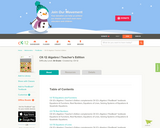Conditional Remix & Share Permitted
CC BY-NC-SA
Rating
0.0 stars

CK-12 Foundation's Algebra I Teacher's Edition FlexBook complements CK-12's Algebra I Student Edition. The solution and assessment guides are available upon request.

Subject:
Mathematics
Material Type:
Textbook
Provider:
CK-12 Foundation
Provider Set:
CK-12 FlexBook
Author:
Fay-Zenk, Mary
McFarland, Andrew
11/06/2009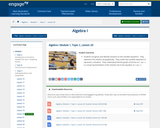Conditional Remix & Share Permitted
CC BY-NC-SA
Rating
0.0 stars

Students recognize and identify solutions to two-variable equations. They represent the solution set graphically. They create two variable equations to represent a situation. They understand that the graph of the line ax + by = c is a visual representation of the solution set to the equation ax + by = c.

Subject:
Math 1
Mathematics
Material Type:
Lesson
Author:
EngageNY
02/02/2020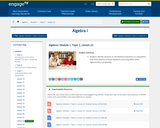Conditional Remix & Share Permitted
CC BY-NC-SA
Rating
0.0 stars

Students identify solutions to simultaneous equations or inequalities; they solve systems of linear equations and inequalities either algebraically or graphically.

Subject:
Math 1
Mathematics
Material Type:
Lesson
Author:
EngageNY
02/02/2020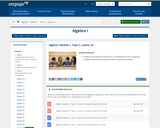Conditional Remix & Share Permitted
CC BY-NC-SA
Rating
0.0 stars

Students use systems of equations or inequalities to solve contextual problems and interpret solutions within a particular context.

Subject:
Math 1
Mathematics
Material Type:
Lesson
Author:
EngageNY
02/02/2020Conditional Remix & Share Permitted
CC BY-NC-SA
Rating
0.0 stars

CK-12 Foundation's Algebra FlexBook is an introduction to algebraic concepts for the high school student. Topics include: Equations & Functions, Real Numbers, Equations of Lines, Solving Systems of Equations & Quadratic Equations.

Subject:
Mathematics
Material Type:
Textbook
Provider:
CK-12 Foundation
Provider Set:
CK-12 FlexBook
12/06/2018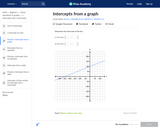Rating
0.0 stars

In this Khan Academy activity, students will determine the intercepts of a line.

Subject:
Mathematics
Material Type:
Activity/Lab
Provider:
08/28/2018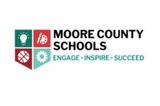Conditional Remix & Share Permitted
CC BY-NC-SA
Rating
0.0 stars

This task explores the real world topic of building light rails. Throughout the implementation of this task the students will learn about the cost of building railways and how to implement them within a budget. This task explores such mathematical concepts of using coordinates to find the distance between points, using coordinates to build polygons and find the area and length of sides, and writing equations of parallel lines.

Subject:
Math 1
Material Type:
Activity/Lab
Author:
Carrie Robledo
James O'Neal
03/26/2021Rating
0.0 stars

Susie is organizing the printing of tickets for a show. She has collected prices from several printers. Your task is to use graphs and algebra to advise Susie on how to choose the best printer.

Subject:
Math 1
Math 2
Math 3
Mathematics
Material Type:
Activity/Lab
Assessment
Lesson Plan
Provider:
MARS, Mathematics Assessment Resource Service
Author:
Shell Center Team
02/26/2019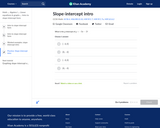Rating
0.0 stars

In this Khan Academy activity, students will practice problems finding the slope, intercepts, and equation of a line.

Subject:
Mathematics
Material Type:
Activity/Lab
Provider:
08/29/2018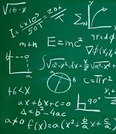Unrestricted Use
CC BY
Rating
0.0 stars

Items that I have used when teaching this unit.&nbsp;

Subject:
Math 1
Material Type:
Unit of Study
Author:
Wendi Hinson
03/23/2020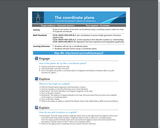Conditional Remix & Share Permitted
CC BY-NC-SA
Rating
0.0 stars

Points on the surface of the earth can be plotted using a coordinate system made from lines of longitude and latitude.

Subject:
Mathematics
Material Type:
Activity/Lab
Author:
ESRI